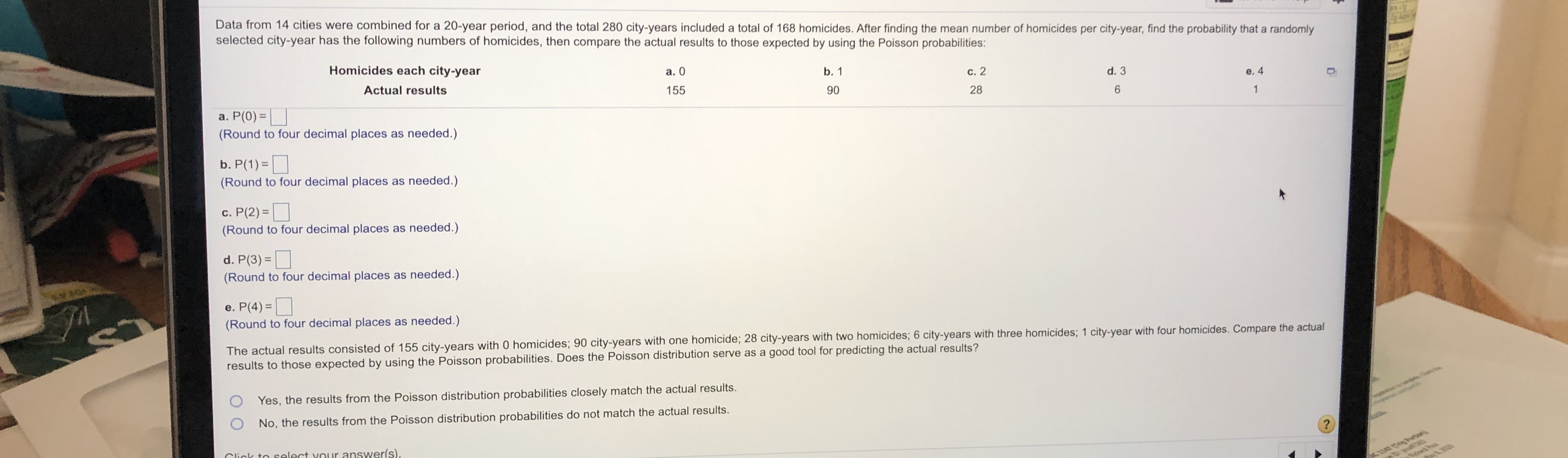# Data from 14 cities were combined for a 20-year period, and the total 280 city-years included a total of 168 homicides. After finding the mean number of homicides per city-year, find the probability that a randomly selected city-year has the following numbers of homicides, then compare the actual results to those expected by using the Poisson probabilities: Homicides each city-year a. 0 c. 2 b. 1 Actual results d. 3 e. 4 155 90 28 a. P(0) = (Round to four decimal places as needed.) b. P(1) =| (Round to four decimal places as needed.) c. P(2) =| (Round to four decimal places as needed.) d. P(3) = (Round to four decimal places as needed.) e. P(4) = (Round to four decimal places as needed.) The actual results consisted of 155 city-years with 0 homicides; 90 city-years with one homicide; 28 city-years with two homicides; 6 city-years with three homicides; 1 city-year with four homicides. Compare the actual results to those expected by using the Poisson probabilities. Does the Poisson distribution serve as a good tool for predicting the actual results? O Yes, the results from the Poisson distribution probabilities closely match the actual results. No, the results from the Poisson distribution probabilities do not match the actual results. Click to select vour answer(s). 1147 (Tg Poroa

Question

Can you help me, please?

(not graded, for acquisition)help_outlineImage TranscriptioncloseData from 14 cities were combined for a 20-year period, and the total 280 city-years included a total of 168 homicides. After finding the mean number of homicides per city-year, find the probability that a randomly selected city-year has the following numbers of homicides, then compare the actual results to those expected by using the Poisson probabilities: Homicides each city-year a. 0 c. 2 b. 1 Actual results d. 3 e. 4 155 90 28 a. P(0) = (Round to four decimal places as needed.) b. P(1) =| (Round to four decimal places as needed.) c. P(2) =| (Round to four decimal places as needed.) d. P(3) = (Round to four decimal places as needed.) e. P(4) = (Round to four decimal places as needed.) The actual results consisted of 155 city-years with 0 homicides; 90 city-years with one homicide; 28 city-years with two homicides; 6 city-years with three homicides; 1 city-year with four homicides. Compare the actual results to those expected by using the Poisson probabilities. Does the Poisson distribution serve as a good tool for predicting the actual results? O Yes, the results from the Poisson distribution probabilities closely match the actual results. No, the results from the Poisson distribution probabilities do not match the actual results. Click to select vour answer(s). 1147 (Tg Poroa fullscreen

## Expert Answer

### Want to see this answer and more?

Experts are waiting 24/7 to provide step-by-step solutions in as fast as 30 minutes!*

*Response times vary by subject and question complexity. Median response time is 34 minutes and may be longer for new subjects.
Tagged in© 2021 bartleby. All Rights Reserved.